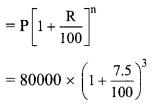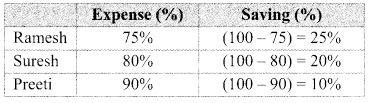# Maharashtra Board Class 9 Maths Solutions Chapter 6 Financial Planning Problem Set 6

## Maharashtra State Board Class 9 Maths Solutions Chapter 6 Financial Planning Problem Set 6

Question 1.
Write the correct alternative answer for each of the following questions.

i. For different types of investments what is the maximum permissible amount under section 80C of income tax ?
(A) ₹ 1,50,000
(B) ₹ 2,50,000
(C) ₹ 1,00,000
(D) ₹ 2,00,000
Answer:
(A) ₹ 1,50,000

ii. A person has earned his income during the financial year 2017-18. Then his assessment year is….
(A) 2016 – 17
(B) 2018 – 19
(C) 2017 – 18
(D) 2015 – 16
Answer:
(B) 2018 – 19

Question 2.
Mr. Shekhar spends 60% of his income. From the balance he donates ₹ 300 to an orphanage. He is then left with ₹ 3,200. What is his income ?
Solution:
Let the income of Shekhar be ₹ x.
Shekhar spends 60% of his income.
∴ Shekhar’s expenditure = 60% of x
∴ Amount remaining with Shekhar = (100 – 60)% of x
= 40% of x
= $$\frac { 1 }{ 2 }$$ × x
= 0.4x
From the balance left, he donates ₹ 300 to an orphanage.
∴ Amount left with Shekhar = 0.4x – 300
Now, the amount left with him is ₹ 3200.
∴ 3200 = 0.4x- 300
∴ 0.4x = 3500∴ The income of Mr. Shekhar is ₹ 8750.

Question 3.
Mr. Hiralal invested ₹ 2,15,000 in a Mutual Fund. He got ₹ 3,05,000 after 2 years. Mr. Ramniklal invested ₹ 1,40,000 at 8% compound interest for 2 years in a bank. Find out the percent gain of each of them. Whose investment was more profitable ?
Solution:
Mr. Hiralal:
Amount invested by Mr. Hiralal in mutual fund = ₹ 2,15,000
Amount received by Mr. Hiralal = ₹ 3,05,000
∴ Mr. Hiralal’s profit = Amount received – Amount invested
= 305000 – 215000 = ₹ 90000
Mr. Hirala’s percentage of profit
= $$\frac { 90000 }{ 215000 }$$ × 100
= 41.86%

Mr. Ramniklal:
P = ₹ 140000, R = 8%, n = 2 years
∴ Compound interest (I)
= A – P= 140000 [(1 + 0.08)2 – 1]
= 140000 [ (1.08)2 – 1]
= 140000(1.1664 – 1)
= 140000 x 0.1664
= ₹ 23296
∴ Mr. Ramniklal’s percentage of profit
= $$\frac { 23296 }{ 140000 }$$ × 100
= 16.64%
∴ The percentage gains of Mr. Hiralal and Mr. Ramniklal are 41.86% and 16.64% respectively, and hence, Mr. Hiralal’s investment was more profitable.

Question 4.
At the start of a year there were ₹ 24,000 in a savings account. After adding ₹ 56,000 to this the entire amount was invested in the bank at 7.5% compound interest. What will be the total amount after 3 years ?
Solution:
Here, P = 24000 + 56000
= ₹ 80000
R = 7.5%, n = 3 years
Total amount after 3 years= 80000 (1 + 0.075)3
= 80000 (1.075)3
= 80000 x 1.242297
= 99383.76
∴ The total amount after 3 years is ₹ 99383.76.

Question 5.
Mr. Manohar gave 20% of his income to his elder son and 30% to his younger son. He gave 10% of the balance income as donation to a school. He still had ₹ 1,80,000 for himself. What was Mr. Manohar’s income ?
Solution:
Let the income of Mr. Manohar be ₹ x.
Amount given to elder son = 20% of x
Amount given to younger son = 30% of x
Total amount given to both sons = (20 + 30)% of x = 50% of x
∴ Amount remaining with Mr. Manohar = (100 – 50)% of x
= 50% of x 50
= $$\frac { 50 }{ 100 }$$ × 100
= 0.5 x
He gave 10% of the balance income as donation to a school.
Amount donated to school = 10% of 0.5x
= $$\frac { 10 }{ 100 }$$ × 0.5x
= 0.05x
∴ Amount remaining with Mr. Manohar after donating to school = 0.5x – 0.05x
= 0.45x
Mr. Manohar still had 1,80,000 for himself after donating to school.
∴ 180000 = 0.45x
∴ $$x=\frac{180000}{0.45}=\frac{180000 \times 100}{0.45 \times 100}=\frac{18000000}{45}=400000$$
∴ The income of Mr. Manoliar is ₹4,00,000.

Question 6.
Kailash used to spend 85% of his income. When his income increased by 36% his expenses also increased by 40% of his earlier expenses. How much percentage of his earning he saves now ?
Solution:
Let the income of Kailash be ₹ x.
Kailash spends 85% of his income.
∴ Kailash’s expenditure = 85% of x
= $$\frac { 85 }{ 100 }$$ × x = 0.85 x
Kailash’s income increased by 36%.
∴ Kailash’s new income = x + 36% of x
= x + $$\frac { 36 }{ 100 }$$ × x
= x + 0.36x
= 1.36x
Kailash’s expenses increased by 40%.
∴ Kailash’s new expenditure = 0.85x + 40% of 0.85x
= 0.85x + $$\frac { 40 }{ 100 }$$ × 0.85 × 100
= 0.85x + 0.4 × 0.85x
= 0.85x (1 + 0.4)
= 0.85x × 1.4
= 1.19x
∴ Kailash’s new saving = Kailash’s new income – Kailash’s new expenditure
= 1.36x – 1.19x
= 0.17x
Percentage of Kailash’s new saving
= $$\frac { 0.17x }{ 1.36x }$$ × 100
= 12.5%
∴ Kailash saves 12.5% of his new earning.

Question 7.
Total income of Ramesh, Suresh and Preeti is ₹ 8,07,000. The percentages of their expenses are 75%, 80% and 90% respectively. If the ratio of their savings is 16 : 17 : 12, then find the annual saving of each of them.
Solution:
Let the annual income of Ramesh, Suresh and Preeti be ₹ x, t y and ₹ z respectively.
Total income of Ramesh, Suresh and Preeti = ₹ 8,07,000
∴ x + y + z = 807000 …(i)∴ Savings of Ramesh = 25% of x
= ₹ $$\frac { 25x }{ 100 }$$ ..(ii)
Savings of Suresh = 20% of y
= ₹$$\frac { 20y }{ 100 }$$ …(iii)
Savings of Preeti = 10% of z
= ₹$$\frac { 10z }{ 100 }$$ …..(iv)

Ratio of their savings = 16 : 17 : 12
Let the common multiple be k.
Savings of Ramesh = ₹ 16 k … (v)
Savings of Suresh = ₹ 17 k … (vi)
Savings of Preeti = ₹ 12 k .. .(vii)∴ z = 120k …(x)
From (i), (viii), (ix) and (x), we get
64k + 85k + 120k = 807000
269k = 807000
k = $$\frac { 807000 }{ 269 }$$
k = 3000
∴ Annual saving of Ramesh = 16k
= 16 x 3000
= ₹ 48,000
Annual saving of Suresh = 17k
= 17 x 3000
= ₹ 51,000
Annual saving of Preeti = 12k
= 12 x 3000
= ₹ 36,000
The annual savings of Ramesh, Suresh and Preeti are ₹ 48,000, ₹ 51,000 and ₹ 36,000 respectively.

Question 8.
Compute the income tax payable by following individuals.
i. Mr. Kadam who is 35 years old and has a taxable income of ₹13,35,000.
ii. Mr. Khan is 65 years of age and his taxable income is ₹4,50,000.
iii. Miss Varsha (Age 26 years) has a taxable income of ₹2,30,000.
Solution:
i. Mr. Kadam is 35 years old and his taxable income is ₹13,35,000.
Mr. Kadam’s income is more than ₹ 10,00,000.
∴ Income tax = ₹1,12,500 + 30% of (taxable income -10,00,000)
= ₹ 1,12,500 + 30% of (13,35,000 – 10,00,000)
= 112500+ $$\frac { 30 }{ 100 }$$ x 335000 100
= 112500+ 100500
= ₹ 213000
Education cess = 2% of income tax
= $$\frac { 2 }{ 100 }$$ x 213000
= ₹ 4260.
Secondary and Higher Education cess
= 1% of income tax
= $$\frac { 1 }{ 100 }$$ x 213000 100
= 2130
Total income tax = Income tax + Education cess + Secondary and higher education cess
= 213000 + 4260 + 2130 = ₹ 2,19,390
∴ Mr. Kadam will have to pay income tax of ₹ 2,19,390.

ii. Mr. Khan is 65 years old and his taxable income is ₹ 4,50,000.
Mr. Khan’s income falls in the slab ₹ 3,00,001 to ₹ 5,00,000.
∴ Income tax
= 5% of (taxable income – 300000)
= 5% of (450000 – 300000)
= $$\frac { 5 }{ 100 }$$ x 150000 100
= ₹ 7500
Education cess = 2% of income tax
= $$\frac { 2 }{ 100 }$$ x 7500
= ₹ 150
Secondary and Higher Education cess = 1 % of income tax
= $$\frac { 1 }{ 100 }$$ x 7500
= 75
Total income tax = Income tax + Education cess + Secondary and higher education cess
= 7500+ 150 + 75
= ₹ 7725
Mr. Khan will have to pay income tax of ₹7725.

iii. Taxable income = ₹2,30,000
age = 26 years
The yearly income of Miss Varsha is less than ₹ 2,50,000.
Hence, Miss Varsha will not have to pay income tax.

Maharashtra Board Class 9 Maths Chapter 6 Financial Planning Problem Set 6 Intext Questions and Activities

Question 1.
With your parent’s help write down the income and expenditure of your family for one week. Make 7 columns for the seven days of the week. Write all expenditure under such heads as provisions, education, medical expenses, travel, clothes and miscellaneous. On the credit side write the amount received for daily expenses, previous balance and any other new income. (Textbook pg. no. 98)

Question 2.
In the holidays, write the accounts for the whole month. (Textbook pg. no. 98)

Question 3.
What is a tax? Which are different types of taxes? Find out more information on following websites
www.incometaxindia.gov.in,
www.mahavat.gov.in
www.gst.gov.in (Textbook pg. no. 99)

Question 4.
Obtain more information about different types of taxes from employees and professionals who pay taxes. (Textbook pg. no. 99)

Question 5.
Obtain information about sections 80C, 80G, 80D of the Income Tax Act. (Textbook pg. no. 103)

Question 6.
Study a PAN card and make a note of all the information it contains. (Textbook pg.no. 103)

Question 7.
Obtain information about all the devices and means used for carrying out cash minus transactions. (Textbook pg, no, 103)

Question 8.
Visit www.incometaxindia.gov.in which is a website of the Government of India. Click on the ‘incometax calculator’ menu. Fill in the form that gets downloaded using an imaginary income and imaginary deductible amounts and try to compute the income tax payable for this income. (Textbook pg.no. 107)
[Students should attempt the above activities on their own.]

Scroll to Top